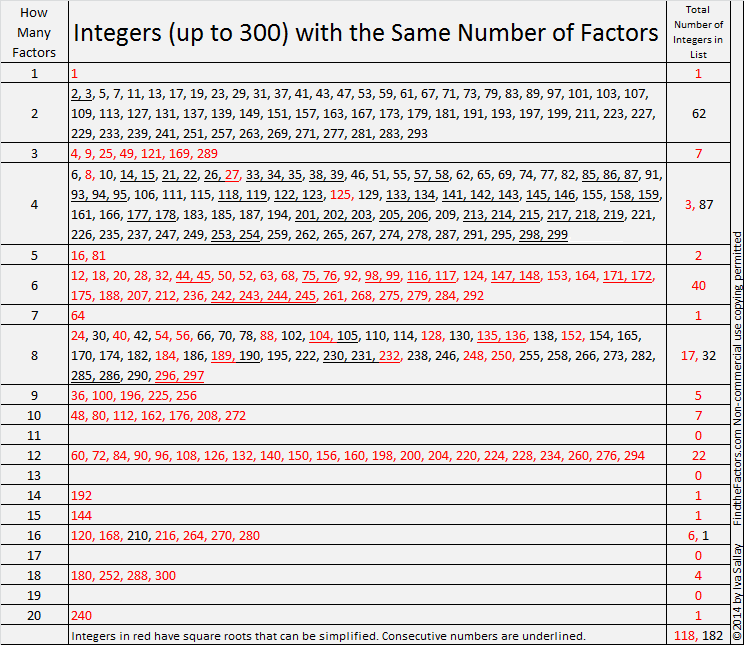# Integers (up to 300) with the Same Number of Factors

• 300 is a composite number.
• Prime factorization: 300 = 2 x 2 x 3 x 5 x 5, which can be written 300 = (2^2) x 3 x (5^2)
• The exponents in the prime factorization are 2, 1 and 2. Adding one to each and multiplying we get (2 + 1)(1 + 1)(2 + 1) = 3 x 2 x 3 = 18. Therefore 300 has exactly 18 factors.
• Factors of 300: 1, 2, 3, 4, 5, 6, 10, 12, 15, 20, 25, 30, 50, 60, 75, 100, 150, 300
• Factor pairs: 300 = 1 x 300, 2 x 150, 3 x 100, 4 x 75, 5 x 60, 6 x 50, 10 x 30, 12 x 25 or 15 x 20
• Taking the factor pair with the largest square number factor, we get √300 = (√3)(√100) = 10√3 ≈ 17.321I made a couple of graphics a few weeks ago anticipating my 300th post.Observations:

• The only number with exactly one factor is one.
• The number of factors of all the black integers on the chart are powers of 2. These integers have irreducible square roots.
• Consecutive integers with the same number of factors can only occur if the number of factors is NOT a prime number.
• 90/300 or 30% of the first 300 integers have 4 factors. (Largest group)
• 62/300 or 20.6666% of the first 300 integers are prime numbers and therefore have 2 factors. (2nd largest group)
• 49/300 or 16.3333% of the first 300 integers have 8 factors. (3rd largest group)
• 40/300 or 13.3333% of the first 300 integers have 6 factors. (4th largest group) All integers with 6 factors have reducible square roots.
• 22/300 or 7.3333% of the first 300 integers have 12 factors. (5th largest group)
• All but 37/300 or 12.3333% of the first 300 integers are in one of those 5 groups.
• 118/300 or 39.3333% of the first 300 integers have square roots that can be simplified.

Warning: the next chart and observations could make your brain hurt:• How many numbers have exactly 2 factors? Euclid proved that there is an infinite number of prime numbers which means there is an infinite number of integers with exactly 2 factors.
• How many integers have exactly 19 factors? Even though the smallest integer with exactly 19 factors is 262,144, there is still an infinite number of integers with exactly that many factors. The integers in that list are each prime number raised to the 18th power. Since there is an infinite number of prime numbers, there is an infinite number of integers with exactly 19 factors.
• {2⁹⁹⁶, 3⁹⁹⁶, 5⁹⁹⁶, . . . } is the infinite list of integers with exactly 997 factors. Likewise {2ᵖ⁻¹, 3ᵖ⁻¹, 5ᵖ⁻¹, . . . } where p is a prime number is the infinite list of integers with exactly p factors.
• If the number of factors is c, a composite number, then it could be said that there is more than an infinite number of integers with that many factors because the infinite list of integers will include {2ᶜ⁻¹, 3ᶜ⁻¹, 5ᶜ⁻¹, . . . } as well as many other integers.

This site uses Akismet to reduce spam. Learn how your comment data is processed.